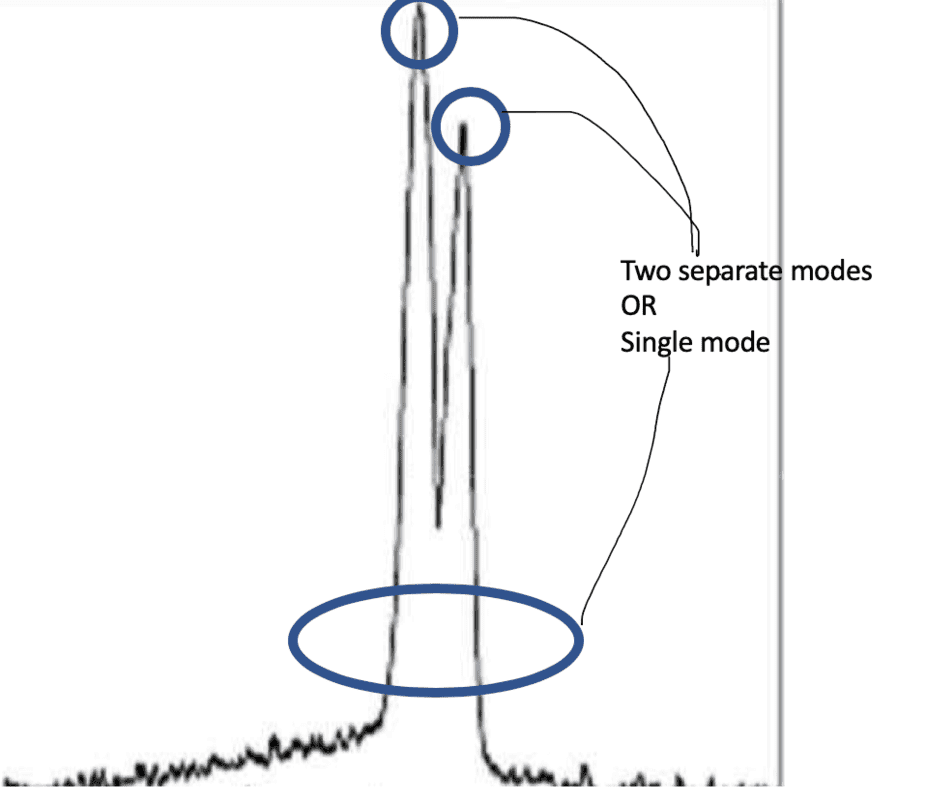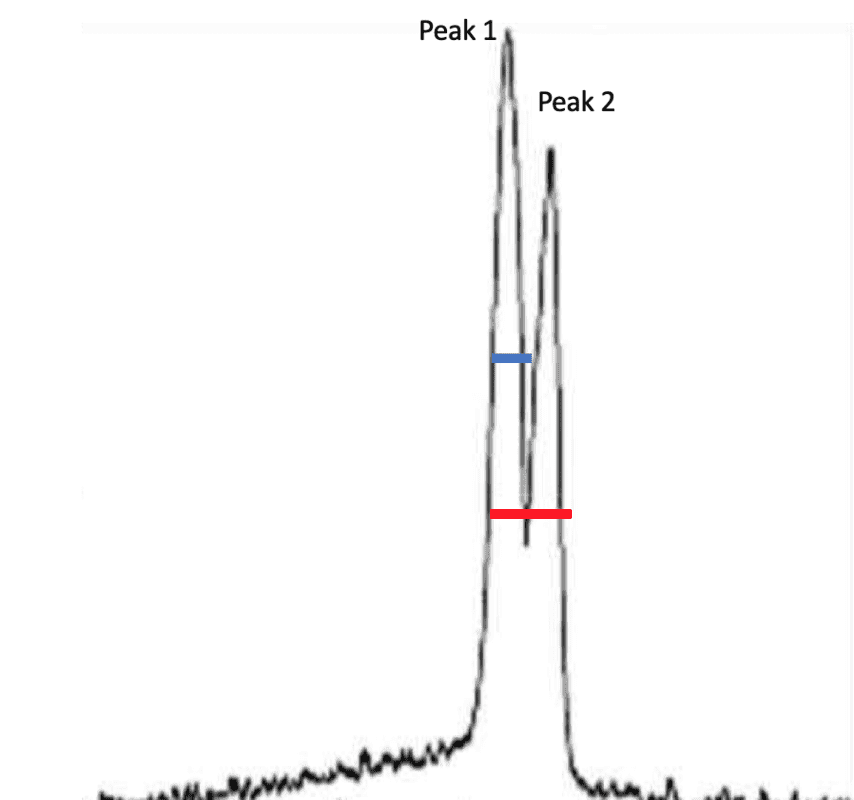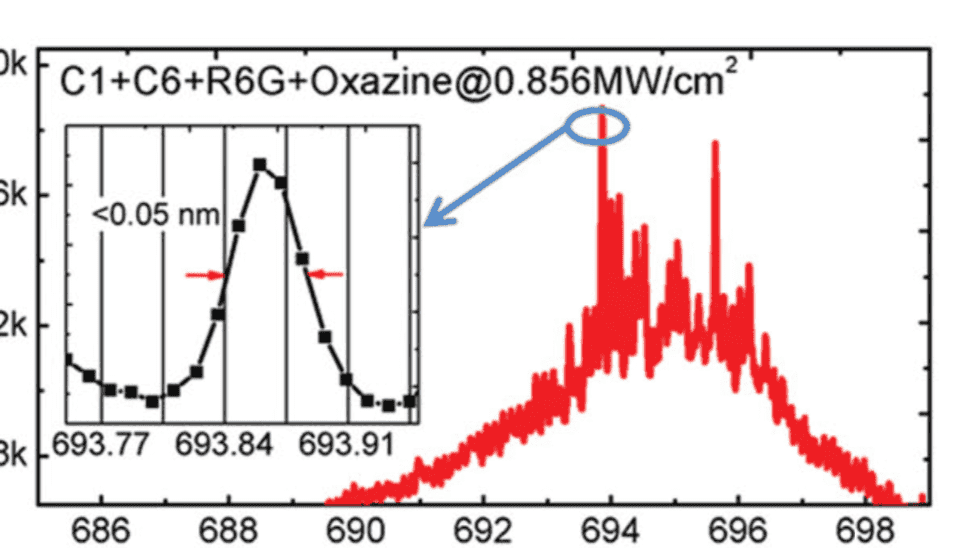# Modes and Q-factor(s) of a random laser

samchan
Many papers about random lasers mention the Q-factor of random lasers. Since a random laser has multiple peaks close to each other like shown in the figure. Does each of these peaks correspond to a unique random lasing mode, or is it just a single mode?Similarly what is the right way to calculate the Q-factor of this random laser (example plot shown below) : Should it be wavelength at Peak1 divided by FWHM at (blue line) or wavelength of Peak1 divided by FWHM at (red line) ?Should the Q-factor be calculated at -3db or FWHM?

•